# LINEAR EQUATIONS DAY 2 SOLVING LINEAR EQUATIONS GROUPS

• Slides: 10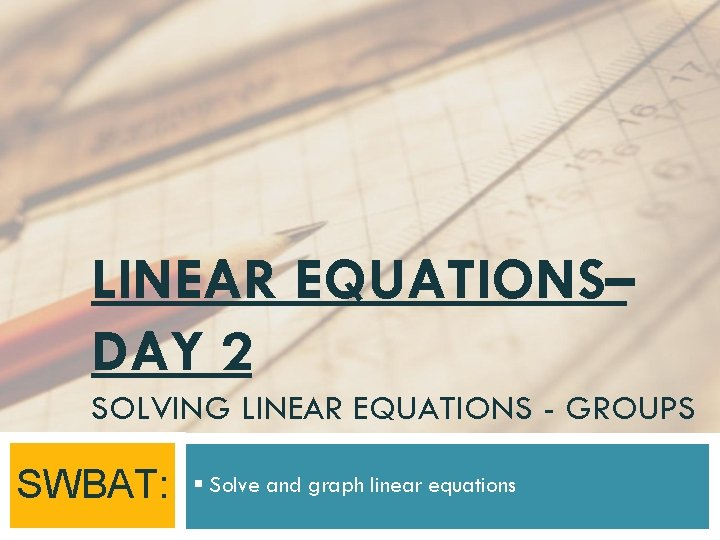LINEAR EQUATIONS– DAY 2 SOLVING LINEAR EQUATIONS - GROUPS SWBAT: § Solve and graph linear equations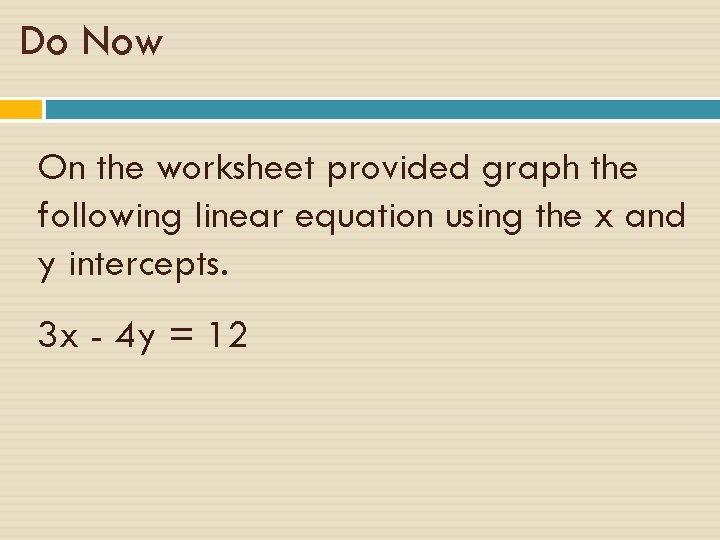Do Now On the worksheet provided graph the following linear equation using the x and y intercepts. 3 x - 4 y = 12Take out the worksheet that we were working on yesterday. We will discuss what problems we had yesterday.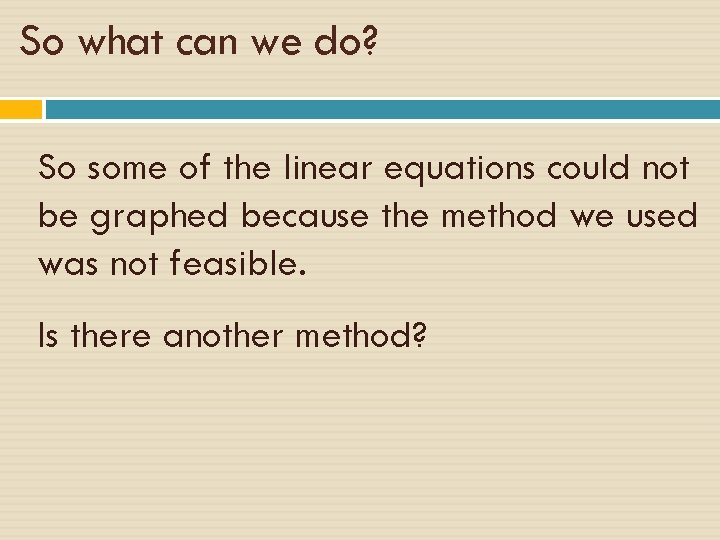So what can we do? So some of the linear equations could not be graphed because the method we used was not feasible. Is there another method?Standard Form vs Slope Intercept Form ØStandard form: Ø Ax + By = C (where a, b, c are integers >1) ØSlope Intercept Form: Ø y = mx + b (where m is the slope and b is the y intercept)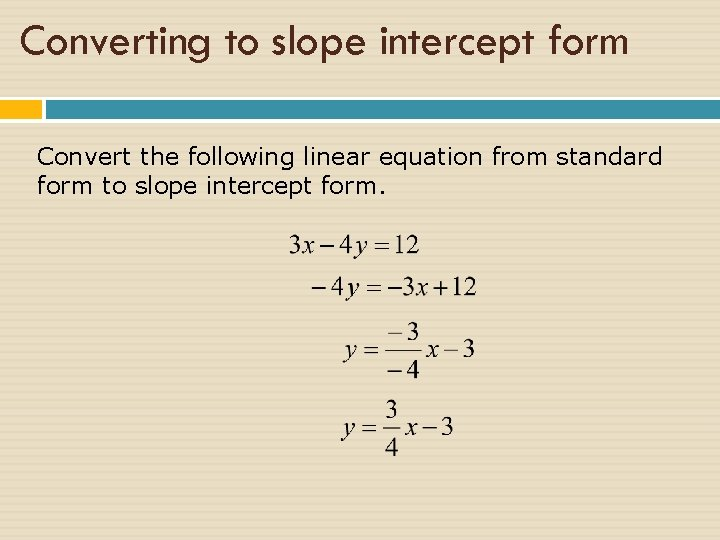Converting to slope intercept form Convert the following linear equation from standard form to slope intercept form.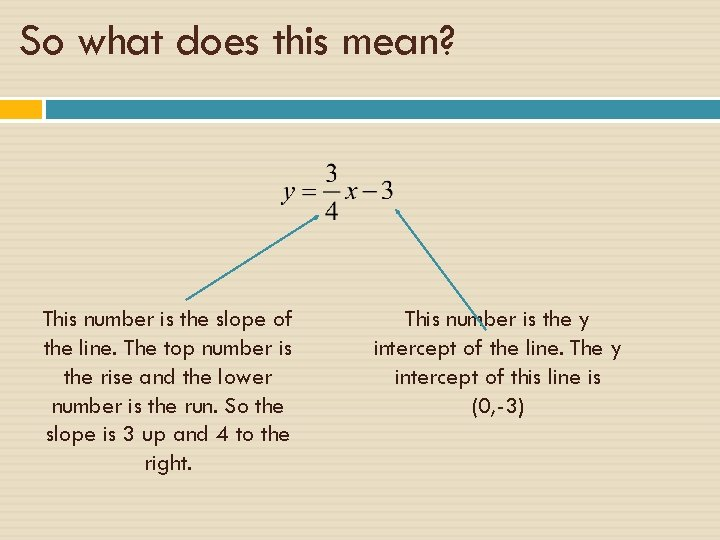So what does this mean? This number is the slope of the line. The top number is the rise and the lower number is the run. So the slope is 3 up and 4 to the right. This number is the y intercept of the line. The y intercept of this line is (0, -3)How do we graph the line? 1. Start with the y intercept, we will graph the point (0, -3) 2. Then use the slope to find the second point to draw your line. 3. Draw the lineApply what you learned In your groups, finish the problems you were assigned. Decide if you should graph the equation by graphing the x and y intercepts OR by using the method you learned today.